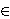### Matija Cencelj, Jerzy Dydak and Aleš Vavpetič

Pedagoška fakulteta, Univerza v Ljubljani, Kardeljeva pl. 16, SI-1111 Ljubljana, Slovenija
e-mail: matija.cencelj@guest.arnes.si

University of Tennessee, Knoxville, TN 37996, USA
e-mail: dydak@math.utk.edu

Fakulteta za Matematiko in Fiziko, Univerza v Ljubljani, Jadranska ulica 19, SI-1111 Ljubljana, Slovenija
e-mail: ales.vavpetic@fmf.uni-lj.si

Abstract.   The purpose of this note is to characterize the asymptotic dimension asdim(X) of metric spaces X in terms similar to Property A of Guoliang Yu. We prove that for a metric space (X,d) and n≥ 0 the following conditions are equivalent:

1. asdim(X,d)≤ n.
2. For each R,ε > 0 there is S > 0 and finite non-empty subsets Ax⊂ B(x,S)× N, xX, such that |AxΔ Ay| / |Ax∩ Ay| < ε if d(x,y) < R and the projection of Ax onto X contains at most n+1 elements for all xX.
3. For each R > 0 there is S > 0 and finite non-empty subsets Ax⊂ B(x,S)× N, xX, such that |AxΔ Ay| / |Ax∩ Ay| < 1/(n+1) if d(x,y) < R and the projection of Ax onto X contains at most n+1 elements for all xX.

2010 Mathematics Subject Classification.   54F45, 55M10, 54C65.

Key words and phrases.   Asymptotic dimension, Property A.

Full text (PDF) (free access)

DOI: 10.3336/gm.47.2.17

References:

1. M. Gromov, Asymptotic invariants of infinite groups, in: Geometric group theory, vol. 2 (G. Niblo and M. Roller, eds.), Cambridge University Press, Cambridge, 1993, 1-295.
MathSciNet

2. N. Higson and J. Roe, Amenable group actions and the Novikov conjecture, J. Reine Angew. Math. 519 (2000), 143-153.
MathSciNet     CrossRef

3. P. Nowak and G. Yu, What is ... property A?, Notices Amer. Math. Soc. 55 (2008), 474-475.
MathSciNet

4. J. Roe, Lectures on coarse geometry, AMS, Providence, 2003.
MathSciNet

5. R. Willett, Some notes on Property A, in: Limits of graphs in group theory and computer science, EPFL Press, Lausanne, 2009, 191-281.
MathSciNet

6. G. Yu, The coarse Baum-Connes conjecture for spaces which admit a uniform embedding into Hilbert space, Invent. Math. 139 (2000), 201-240.
MathSciNet     CrossRef